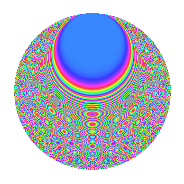# Properties

 Label 160.6.xLevel 160 Weight 6 Character orbit x Rep. character $$\chi_{160}(21,\cdot)$$ Character field $$\Q(\zeta_{8})$$ Dimension 320 Sturm bound 144

# Related objects

## Defining parameters

 Level: $$N$$ $$=$$ $$160 = 2^{5} \cdot 5$$ Weight: $$k$$ $$=$$ $$6$$ Character orbit: $$[\chi]$$ $$=$$ 160.x (of order $$8$$ and degree $$4$$) Character conductor: $$\operatorname{cond}(\chi)$$ $$=$$ $$32$$ Character field: $$\Q(\zeta_{8})$$ Sturm bound: $$144$$

## Dimensions

The following table gives the dimensions of various subspaces of $$M_{6}(160, [\chi])$$.

Total New Old
Modular forms 488 320 168
Cusp forms 472 320 152
Eisenstein series 16 0 16

## Trace form

 $$320q + O(q^{10})$$ $$320q + 200q^{10} - 2480q^{14} - 8360q^{16} + 3240q^{18} + 7600q^{20} + 13592q^{22} - 3344q^{23} - 32480q^{24} + 14928q^{27} + 4360q^{28} + 37160q^{32} + 12760q^{34} - 68856q^{36} - 69640q^{38} + 89808q^{39} + 107240q^{42} - 64144q^{43} - 7440q^{44} - 126656q^{46} + 20880q^{51} - 36944q^{52} + 98912q^{53} + 114008q^{54} + 291320q^{56} - 25992q^{58} + 28960q^{59} - 96160q^{61} - 127224q^{62} + 317520q^{63} + 187440q^{64} + 144880q^{66} + 122320q^{67} + 7152q^{68} + 44640q^{69} - 143840q^{71} - 414072q^{72} - 53960q^{74} - 256000q^{76} + 29792q^{77} + 722328q^{78} + 96800q^{80} + 658480q^{83} - 492520q^{84} - 630824q^{86} - 223280q^{88} + 142200q^{90} - 400224q^{91} - 313192q^{92} - 452792q^{94} - 577600q^{95} + 910184q^{96} + 1602480q^{98} - 339520q^{99} + O(q^{100})$$

## Decomposition of $$S_{6}^{\mathrm{new}}(160, [\chi])$$ into newform subspaces

The newforms in this space have not yet been added to the LMFDB.

## Decomposition of $$S_{6}^{\mathrm{old}}(160, [\chi])$$ into lower level spaces

$$S_{6}^{\mathrm{old}}(160, [\chi]) \cong$$ $$S_{6}^{\mathrm{new}}(32, [\chi])$$$$^{\oplus 2}$$

## Hecke characteristic polynomials

There are no characteristic polynomials of Hecke operators in the database